# Basic probability

## Basic Probability Definition

What is probability theory? A branch of mathematics concerned with the analysis of random phenomena. The outcome of a random event cannot be determined before it occurs, but it may be any one of several possible outcomes. The actual outcome is considered to be determined by chance.

## Fundamental Terms

2.

Continuous Random Variable is one which takes an infinite number of possible values. Continuous random variables are usually measurements.

A simple example is the tossing of a fair (unbiased) coin. Since the coin is fair, the two outcomes (“heads” and “tails”) are both equally probable; the probability of “heads” equals the probability of “tails”; and since no other outcomes are possible, the probability of either “heads” or “tails” is 1/2 (which could also be written as 0.5 or 50%).

Basic Probability Formulas

0 ≤ P(A) ≤ 1

#### Rule of Complementary Events

P(AC) + P(A) = 1

P(A∪B) = P(A) + P(B) - P(A∩B)

#### Disjoint Events

Events A and B are disjoint iff

P(A∩B) = 0

#### Conditional Probability

P(A | B) = P(A∩B) / P(B)

#### Bayes Formula

P(A | B) = P(B | A) ⋅ P(A) / P(B)

#### Independent Events

Events A and B are independent iff

P(A∩B) = P(A) ⋅ P(B)

FX(x) = P(Xx)

#### Probability Mass Function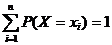#### Probability Density Function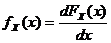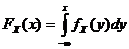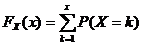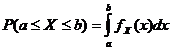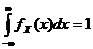#### Covariance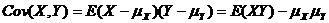#### Correlation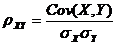Bernoulli: 0-failure 1-success

Geometric: 0-failure 1-success

Hypergeometric: N objects with K success objects, n objects are taken.

Currently, we have around 1975 calculators, conversion tables and usefull online tools and software features for students, teaching and teachers, designers and simply for everyone.